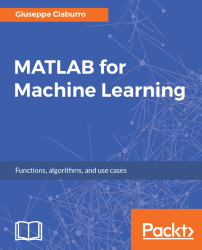•#### MATLAB for Machine Learning#### Overview of this book

MATLAB is the language of choice for many researchers and mathematics experts for machine learning. This book will help you build a foundation in machine learning using MATLAB for beginners. You’ll start by getting your system ready with t he MATLAB environment for machine learning and you’ll see how to easily interact with the Matlab workspace. We’ll then move on to data cleansing, mining and analyzing various data types in machine learning and you’ll see how to display data values on a plot. Next, you’ll get to know about the different types of regression techniques and how to apply them to your data using the MATLAB functions. You’ll understand the basic concepts of neural networks and perform data fitting, pattern recognition, and clustering analysis. Finally, you’ll explore feature selection and extraction techniques for dimensionality reduction for performance improvement. At the end of the book, you will learn to put it all together into real-world cases covering major machine learning algorithms and be comfortable in performing machine learning with MATLAB.
Title PageCreditsForewordwww.PacktPub.comCustomer FeedbackPrefaceFree Chapter
Getting Started with MATLAB Machine LearningImporting and Organizing Data in MATLABFrom Data to Knowledge DiscoveryFinding Relationships between Variables - Regression TechniquesPattern Recognition through Classification AlgorithmsIdentifying Groups of Data Using Clustering MethodsSimulation of Human Thinking - Artificial Neural NetworksImproving the Performance of the Machine Learning Model - Dimensionality ReductionMachine Learning in Practice## Chapter 9. Machine Learning in Practice

In Chapter 1, Getting Started with MATLAB Machine Learning, we said that machine learning is based on the concept of learning by example--knowledge gained by starting from a set of positive examples or instances of the concept to be learned, and negative examples or non-instances of the concept. In this regard, let's not forget that this concept is borrowed from the human experience, which has always been based on learning on analysis through the series of examples.

After analyzing in detail the different machine learning algorithms, it is time to put them to practice. This last chapter is meant to be a short reference, covering some of the major machine learning algorithms. In this chapter, you will just apply what has been learned. You can explore regression, classification, and clustering algorithms applied to datasets resulting from real cases. The basic concepts we have learned from the previous chapters, we will now put them into practice. If...